# Determining distances through the moving cluster method

There is another method that can help confirm the results of the parallax method. (This method is not included in our book, but it is quite ingenious.) It works for stars that are further away if they are in a cluster. It is necessary to suppose that the stars are all moving together. This is is based on the idea that the stars in the cluster all came from the same gas cloud.

This method has been successfully applied to (just) one cluster, the Hyades (the head of Taurus the Bull), which is found to be 40 pc away.

To understand the method, we need to proceed step by step. We consider first one star in the cluster. Let d be the distance to the star in pc. We don't know d. We want to know it.

From photographs taken, say, 10 years apart, we can see that the star has moved. (It has proper motion.) Let us suppose that the proper motion is 3 arc sec in ten years.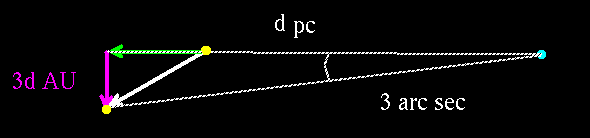We can conclude that the star has moved 3 times d astronomical units across our line of sight. (But we don't know d).

From measurments of the doppler shift of light from our star, we can determine how fast it is moving toward us or away from us. Let us suppose that the speed is 2 AU per year away from us, so that in the ten years it has moved 20 AU further away: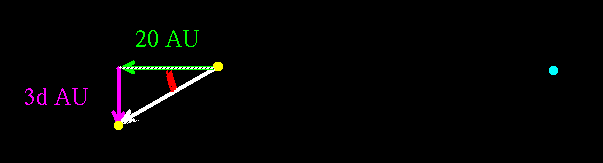Now if we knew the direction in which the star is moving, we could determine d.

How can we determine the direction of motion? Here is where the cluster comes in. Our star is in a cluster and they are all moving in the same direction. (That is, we have to assume that they are all moving in the same direction.).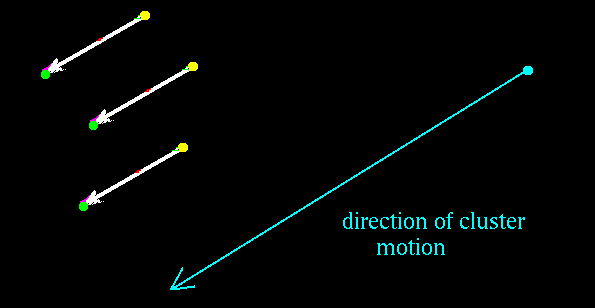If there were some way that we could determine the direction in which the cluster is moving, we would be done!

## The art of finding the direction of the cluster

Here is a famous surrealistic painting by Magritte. It may be surrealistic, but part of it is realistic. That has to do with how parallel lines in three dimensional space look when represented on a two dimensional canvas. Here is what Magritte did.

All artists know this, and astronomers know it too. From our two photographs of the star cluster taken 10 years apart, we can plot the directions that the stars are moving as seen on the ``canvas'' of the sky.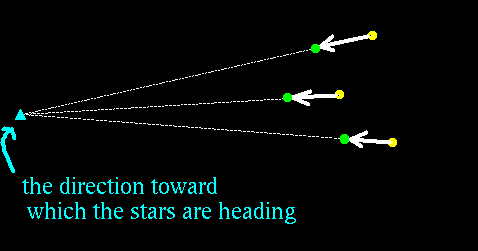Since the paths of the stars are parallel lines, they seem to converge on a vanishing point. The direction from Earth to the vanishing point is the direction of motion of the cluster.

We use the direction of motion that we now know to determine the distance to the stars in the cluster.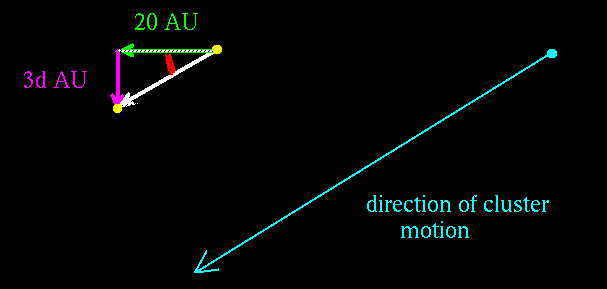Astronomers use trigonometry, but you could just make a scale drawing to determine the length of the purple line in AU. Dividing this length by 3 in our example gives the distance to the cluster in parsecs.

Updated 22 Octobber 2007

Davison E. Soper, Institute of Theoretical Science, University of Oregon, Eugene OR 97403 USA